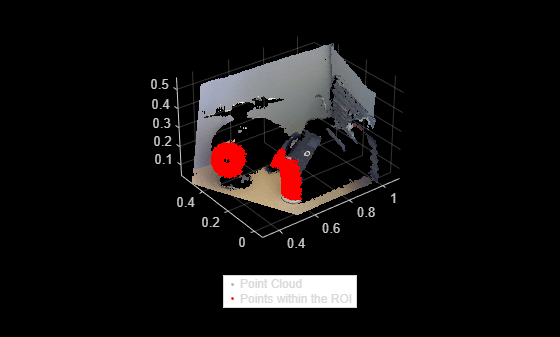# findPointsInROI

Find points within a region of interest in the point cloud

## Syntax

``indices = findPointsInROI(ptCloud,roi)``
``indices = findpointsInROI(ptCloud,roi,camMatrix)``

## Description

example

````indices = findPointsInROI(ptCloud,roi)` returns the points within a region of interest (ROI) in the input point cloud. The points within the specified ROI are obtained using Kd-tree based search algorithm.```

example

````indices = findpointsInROI(ptCloud,roi,camMatrix)` returns the points within a ROI in the input point cloud. The input point cloud is an organized point cloud data generated by a depth camera. The points within the specified ROI are obtained using fast approximate neighbor search algorithm. The function uses the camera projection matrix `camMatrix` to know the relationship between adjacent points and hence, speeds up the search. However, the results have lower accuracy as compared to the Kd-tree based approach. NoteThis function only supports organized point cloud data produced by RGB-D sensors.You can use `estimateCameraMatrix` to estimate camera projection matrix for the given point cloud data. ```

## Examples

collapse all

Read a point cloud data into the workspace.

`ptCloud = pcread('teapot.ply');`

Define a cuboid ROI within the range of the x, y and z coordinates of the input point cloud.

`roi = [-2 2 -2 2 2.4 3.5];`

Find the indices of the points that lie within the cuboid ROI.

`indices = findPointsInROI(ptCloud,roi);`

Select the points that lie within the cuboid ROI and store as a point cloud object.

`ptCloudB = select(ptCloud,indices);`

Display the input point cloud and the point cloud within the specified ROI.

```figure pcshow(ptCloud.Location,[0.5 0.5 0.5]) hold on pcshow(ptCloudB.Location,'r'); legend('Point Cloud','Points within ROI','Location','southoutside','Color',[1 1 1]) hold off```Find points within a cuboid ROI in the organized point cloud data by using the camera projection matrix. Compute the camera projection matrix from sampled point cloud data points and their corresponding image point coordinates.

Load an organized point cloud data into the workspace. The point cloud is generated by using the Kinect depth sensor.

```ld = load('object3d.mat'); ptCloud = ld.ptCloud;```

Specify the step size for sampling the point cloud data.

`stepSize = 100;`

Sample the input point cloud and store the sampled 3-D point coordinates as a point cloud object.

```indices = 1:stepSize:ptCloud.Count; tempPtCloud = select(ptCloud,indices);```

Remove invalid points from the sampled point cloud.

`[tempPtCloud,validIndices] = removeInvalidPoints(tempPtCloud);`

Get the 3-D world point coordinates from input point cloud.

`worldPoints = tempPtCloud.Location;`

Find the 2-D image coordinates corresponding to the 3-D point coordinates of input point cloud.

```[Y,X] = ind2sub([size(ptCloud.Location,1),size(ptCloud.Location,2)],indices); imagePoints = [X(validIndices)' Y(validIndices)'];```

Estimate camera projection matrix from the image and the world point coordinates.

`camMatrix = estimateCameraMatrix(imagePoints,worldPoints);`

Specify a cuboid ROI within the range of the x, y and z coordinates of the input point cloud.

`roi = [0.3 0.7 0 0.4 0.1 0.3];`

Find the indices of the point cloud data that lie within the cuboid ROI.

`indices = findPointsInROI(ptCloud,roi);`

Use the point cloud method `select` to get the point cloud data of points within the ROI.

`ptCloudB = select(ptCloud,indices);`

Display the input point cloud and the points within the cuboid ROI.

```figure pcshow(ptCloud) hold on pcshow(ptCloudB.Location,'r'); legend('Point Cloud','Points within the ROI','Location','southoutside','Color',[1 1 1]) hold off```## Input Arguments

collapse all

Point cloud, specified as a `pointCloud` object.

Region of interest, specified as a six-element vector of form [xmin, xmax, ymin, ymax, zmin, zmax], where:

• xmin and xmax are the minimum and the maximum limits along the x-axis respectively.

• ymin and ymax are the minimum and the maximum limits along the y-axis respectively.

• zmin and zmax are the minimum and the maximum limits along the z-axis respectively.

Camera projection matrix, specified as a 4-by-3 matrix that maps 3-D world points to 2-D image points. You can find `camMatrix` by using the `estimateCameraMatrix` function.

## Output Arguments

collapse all

Indices of stored points, returned as a column vector. The vector contains the linear indices of the ROI points stored in the point cloud.

 Muja, M. and David G. Lowe. "Fast Approximate Nearest Neighbors with Automatic Algorithm Configuration". In VISAPP International Conference on Computer Vision Theory and Applications. 2009. pp. 331–340.